Mathematics
Easy

Question

# The pair of fractions among the following that are not equivalent is

##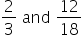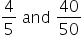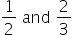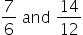Hint:

## The correct answer is:### a.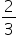and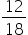2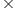18           123= 36                = 36As the cross multiplication of both fractions are equal. So its an equivalent fraction.b.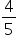and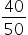4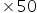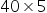= 200             = 200As the cross multiplication of both fraction are equal. So its an equivalent fraction.c.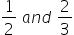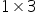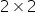= 3              = 4as the cross multiplication of both fractions are not equal. So its not an equivalent fraction.d.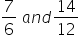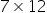14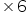= 84           = 84as the cross multiplication of both fractions are equal. So its an equivalent fraction.Therefore the pair of fraction which is not an equivalent fraction is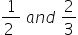.#### With Turito Foundation.#### Get an Expert Advice From Turito.